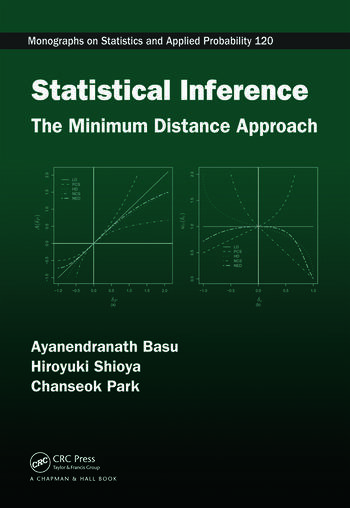# Statistical Inference: The Minimum Distance Approach

## 1st Edition

Ayanendranath Basu, Hiroyuki Shioya, Chanseok Park

Chapman and Hall/CRC
Published June 22, 2011
Reference - 429 Pages - 47 B/W Illustrations
ISBN 9781420099652 - CAT# K10008
Series: Chapman & Hall/CRC Monographs on Statistics and Applied Probability

was \$120.00

USD\$96.00

SAVE ~\$24.00

FREE Standard Shipping!

## Preview

### Summary

In many ways, estimation by an appropriate minimum distance method is one of the most natural ideas in statistics. However, there are many different ways of constructing an appropriate distance between the data and the model: the scope of study referred to by "Minimum Distance Estimation" is literally huge. Filling a statistical resource gap, Statistical Inference: The Minimum Distance Approach comprehensively overviews developments in density-based minimum distance inference for independently and identically distributed data. Extensions to other more complex models are also discussed.

Comprehensively covering the basics and applications of minimum distance inference, this book introduces and discusses:

• The estimation and hypothesis testing problems for both discrete and continuous models
• The robustness properties and the structural geometry of the minimum distance methods
• The inlier problem and its possible solutions, and the weighted likelihood estimation problem
• The extension of the minimum distance methodology in interdisciplinary areas, such as neural networks and fuzzy sets, as well as specialized models and problems, including semi-parametric problems, mixture models, grouped data problems, and survival analysis.

Statistical Inference: The Minimum Distance Approach gives a thorough account of density-based minimum distance methods and their use in statistical inference. It covers statistical distances, density-based minimum distance methods, discrete and continuous models, asymptotic distributions, robustness, computational issues, residual adjustment functions, graphical descriptions of robustness, penalized and combined distances, weighted likelihood, and multinomial goodness-of-fit tests. This carefully crafted resource is useful to researchers and scientists within and outside the statistics arena.